### PROC MI Statement

PROC MI <options> ;

The PROC MI statement invokes the MI procedure. Table 57.1 summarizes the options available in the PROC MI statement.

Table 57.1: Summary of PROC MI Options

Option

Description

Data Sets

Specifies the input data set

Specifies the output data set with imputed values

Imputation Details

Specifies the number of imputations

Specifies the seed to begin random number generator

Specifies units to round imputed variable values

Specifies maximum values for imputed variable values

Specifies minimum values for imputed variable values

Specifies the maximum number of iterations to impute values in the specified range

Specifies the singularity criterion

Statistical Analysis

Specifies the level for the confidence interval,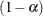Specifies means under the null hypothesis

Printed Output

Suppresses all displayed output

Displays univariate statistics and correlations

The following options can be used in the PROC MI statement. They are listed in alphabetical order.

ALPHA=specifies that confidence limits be constructed for the mean estimates with confidence level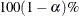, where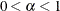. The default is ALPHA=0.05.

DATA=SAS-data-set

names the SAS data set to be analyzed by PROC MI. By default, the procedure uses the most recently created SAS data set.

MAXIMUM=numbers

specifies maximum values for imputed variables. When an intended imputed value is greater than the maximum, PROC MI redraws another value for imputation. If only one number is specified, that number is used for all variables. If more than one number is specified, you must use a VAR statement, and the specified numbers must correspond to variables in the VAR statement. The default number is a missing value, which indicates no restriction on the maximum for the corresponding variable

The MAXIMUM= option is related to the MINIMUM= and ROUND= options, which are used to make the imputed values more consistent with the observed variable values. These options are applicable only if you use the MCMC method or the monotone regression method.

When specifying a maximum for the first variable only, you must also specify a missing value after the maximum. Otherwise, the maximum is used for all variables. For example, the MAXIMUM= 100  . option sets a maximum of 100 for the first analysis variable only and no maximum for the remaining variables. The MAXIMUM= . 100 option sets a maximum of 100 for the second analysis variable only and no maximum for the other variables.

MINIMUM=numbers

specifies the minimum values for imputed variables. When an intended imputed value is less than the minimum, PROC MI redraws another value for imputation. If only one number is specified, that number is used for all variables. If more than one number is specified, you must use a VAR statement, and the specified numbers must correspond to variables in the VAR statement. The default number is a missing value, which indicates no restriction on the minimum for the corresponding variable

MINMAXITER=number

specifies the maximum number of iterations for imputed values to be in the specified range when the option MINIMUM or MAXIMUM is also specified. The default is MINMAXITER=100.

MU0=numbers
THETA0=numbers

specifies the parameter values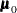under the null hypothesis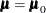for the population means corresponding to the analysis variables. Each hypothesis is tested with a t test. If only one number is specified, that number is used for all variables. If more than one number is specified, you must use a VAR statement, and the specified numbers must correspond to variables in the VAR statement. The default is MU0=0.

If a variable is transformed as specified in a TRANSFORM statement, then the same transformation for that variable is also applied to its corresponding specified MU0= value in the t test. If the parameter valuesfor a transformed variable are not specified, then a value of zero is used for the resultingafter transformation.

NIMPUTE=number

specifies the number of imputations. The default is NIMPUTE=5. You can specify NIMPUTE=0 to skip the imputation. In this case, only tables of model information, missing data patterns, descriptive statistics (SIMPLE option), and MLE from the EM algorithm (EM statement) are displayed.

NOPRINT

suppresses the display of all output. Note that this option temporarily disables the Output Delivery System (ODS); see Chapter 20: Using the Output Delivery System, for more information.

OUT=SAS-data-set

creates an output SAS data set that contains imputation results. The data set includes an index variable, _Imputation_, to identify the imputation number. For each imputation, the data set contains all variables in the input data set with missing values being replaced by the imputed values. See the section Output Data Sets for a description of this data set.

ROUND=numbers

specifies the units to round variables in the imputation. If only one number is specified, that number is used for all continuous variables. If more than one number is specified, you must use a VAR statement, and the specified numbers must correspond to variables in the VAR statement. When the classification variables are listed in the VAR statement, their corresponding roundoff units are not used. The default number is a missing value, which indicates no rounding for imputed variables.

When specifying a roundoff unit for the first variable only, you must also specify a missing value after the roundoff unit. Otherwise, the roundoff unit is used for all variables. For example, the option ROUND= 10  . sets a roundoff unit of 10 for the first analysis variable only and no rounding for the remaining variables. The option ROUND= . 10 sets a roundoff unit of 10 for the second analysis variable only and no rounding for other variables.

The ROUND= option sets the precision of imputed values. For example, with a roundoff unit of 0.001, each value is rounded to the nearest multiple of 0.001. That is, each value has three significant digits after the decimal point. See Example 57.3 for an illustration of this option.

SEED=number

specifies a positive integer to start the pseudo-random number generator. The default is a value generated from reading the time of day from the computer’s clock. However, in order to duplicate the results under identical situations, you must use the same value of the seed explicitly in subsequent runs of the MI procedure.

The seed information is displayed in the Model Information table so that the results can be reproduced by specifying this seed with the SEED= option. You need to specify the same seed number in the future to reproduce the results.

SIMPLE

displays simple descriptive univariate statistics and pairwise correlations from available cases. For a detailed description of these statistics, see the section Descriptive Statistics.

SINGULAR=p

specifies the criterion for determining the singularity of a covariance matrix based on standardized variables, where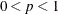. The default is SINGULAR=1E–8.

Suppose that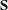is a covariance matrix and v is the number of variables in. Based on the spectral decomposition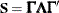, where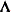is a diagonal matrix of eigenvalues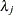,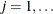, v, where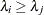when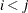, and
is a matrix with the corresponding orthonormal eigenvectors ofas columns,is considered singular when an eigenvalueis less than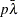, where the average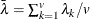.